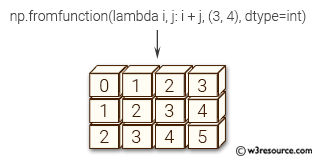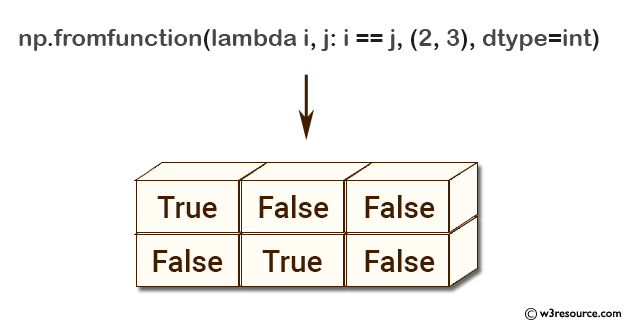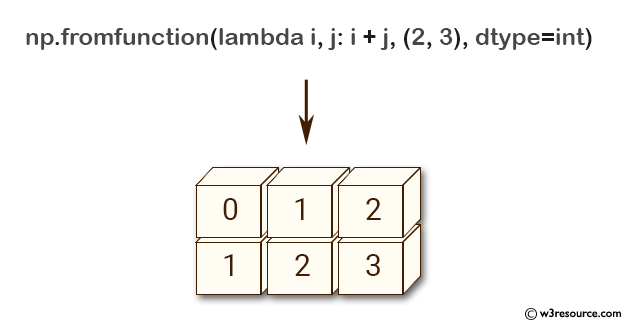# NumPy array creation: fromfunction() function

## fromfunction() function

The fromfunction() function is used to construct an array by executing a function over each coordinate.
The resulting array therefore has a value fn(x, y, z) at coordinate (x, y, z).

Syntax:

`numpy.fromfunction(function, shape, **kwargs)`Version: 1.15.0

Parameter:

Name Description Required /
Optional
function The function is called with N parameters, where N is the rank of shape. Each parameter represents the coordinates of the array varying along a specific axis. For example, if shape were (2, 2), then the parameters would be array([[0, 0], [1, 1]]) and array([[0, 1], [0, 1]]) Required
shape Shape of the output array, which also determines the shape of the coordinate arrays passed to function. Required
dtype Data-type of the coordinate arrays passed to function. By default, dtype is float. Optional

Return value:

fromfunction : any
The result of the call to function is passed back directly. Therefore the shape of fromfunction is completely determined by function. If function returns a scalar value, the shape of fromfunction would match the shape parameter.

Example-1: NumPy.fromfunction() method

``````>>> import numpy as np
>>> np.fromfunction(lambda i, j: i == j, (2, 3), dtype=int)
array([[ True, False, False],
[False,  True, False]], dtype=bool)
``````

Pictorial Presentation:Example-2: NumPy.fromfunction() method

``````>>> import numpy as np
>>> np.fromfunction(lambda i, j: i + j, (2, 3), dtype=int)
array([[0, 1, 2],
[1, 2, 3]])
``````

Pictorial Presentation:Python - Numpy Code Editor:

Previous: copy()
Next: fromiter()

﻿